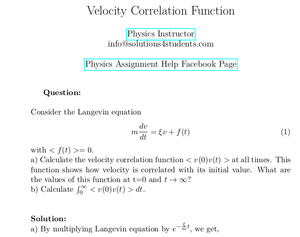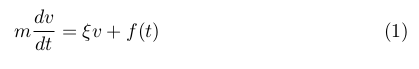Solved Problems in Physics, Physical Chemistry, and Computer Simulation Projects for College and Graduate Students.# Velocity Correlation Function

Regular price
\$3.90
Sale price
\$3.90
Tax included.

Question:
Consider the Langevin equationwith < f (t) >= 0.

a) Calculate the velocity correlation function < v(0)v(t) > at all times. This function shows how velocity is correlated with its initial value. What are the values of this function at t=0 and t → ∞?

b) CalculateBy purchasing this product, you will get the step by step solution of the above problem in pdf format and the corresponding latex file where you can edit the solution. I am also available to help  you with any possible question you may have.